- JoNova - http://joannenova.com.au -

New Science 12: How do we model the thermal inertia of the Earth?

Posted By David Evans On October 16, 2015 @ 4:49 pm In Global Warming | Comments Disabled

Basic models take a top down approach, focusing on gross input and output rather than all the details within the system (which is mainly left to the feedbacks parameter). This makes them very different to the GCMs, which attempt to add up the climate from the bottom up and predict based on adding up grids and guesstimates of clouds, humidity, ice, etc.

The energy coming in to the Earth is called absorbed solar radiation (ASR). It varies significantly. The Earth will absorb the peaks and troughs of this to a certain extent. If we step back and look at the big picture, the question is how many years does it take for a step up in incoming energy to spread its way through the climate system, vanish into the top layer of the ocean, come back out and be released to space. To some extent that extra energy gets absorbed for a while before being released. David analyzed this system from the outside, graphing it like a low pass filter in electronics. (How much “noise” of spikes and troughs in ASR is being smoothed out by the Earth’s climate?)

In a low pass filter graph against frequency, the response of the filter is a flat line at lower frequencies, so all the bumps and wiggles in ASR at those low frequencies are faithfully reproduced in the outgoing longwave radiation (OLR). We know for sure that if the Sun gave off more energy (or somehow indirectly reduced our cloud-cover so that we absorbed more energy) that over a period of say, 200 years, the relationship is one to one. More energy in, equals more energy out. But the question is, how short a period does that apply too? Obviously not say 5 minutes. What kind of time-frame does the low pass filter work on?

The answer is a bit complicated because in every solar cycle (roughly every 11 years) there is a separate effect called the “notch” — the sun churns over it’s magnetic field, releases a little bit more solar radiation — and some effect we haven’t pinned down yet reduces Earth’s sensitivity to that extra bit of incoming radiation. (For those familiar with low pass filter graphs, that means the elbow or bend in the low pass filer graph gets obscured by a separate effect, which is the notch around 11 years.)

In the end, the best we can do is come up with a range, but it’s still useful. The low pass filter effect operates on a similar time-frame to the totally separate notch effect. Ultimately the outer edge of the Earth’s Climate system (including the atmosphere and the land we live on) absorbs, shares, and emits 90% of those spikes and troughs either within about 2 years, or maybe about 8 years, depending on which side of the notch the bend in the low pass filter graph is (the notch obscures the very range we are interested in).

The empirical transfer function and the notch are ahead of us in this series, and for now we are just concerned with modeling the thermal inertia, by describing it with an equation.
– Jo

# 12. Modeling the Thermal Inertia of the Earth

Dr David Evans, 16 October 2015, David Evans’ Basic Climate Models Home, Intro, Previous, Next, Nomenclature.

This post derives the relationship between absorbed solar radiation (ASR) and the radiating temperature, which is at the heart of the solar response in the sum-of-warmings model within the alternative model.

This relationship  is found to have the same structure as that of a simple low pass filter, so the thermal inertia of the Earth is mimicked by a low pass filter. The radiating temperature, which determines the outgoing longwave radiation (OLR), is a smoothed version of the ASR — slow changes in the ASR are faithfully reproduced in the radiating temperature and OLR, but faster changes are attenuated.

The result for transitions between steady states is straightforward and agrees with the conventional basic climate model; the main interest here is the time lag or thermal inertia when not merely modeling the changes between steady states, which is used later in the notch-delay solar model.

### Functions of Time

Unlike the rest of the analyses in this series, in this section of this post we consider climate quantities at any time, rather than just in or between steady states. Let the ASR A, radiating temperature TR, and OLR R be considered as functions of time t, here only.

The only significant source of input energy to the climate system is ASR, because reflected TSI just bounces back out to space without heating the Earth — so the rate of heat input to the Earth, per unit area, is A. The only significant loss of energy is outgoing longwave radiation (OLR) — so the current rate of heat output, per unit area, isby the Stefan-Boltzmann law (Eq. (2) of post 8). The variables we are concerned with here cannot characterize the total amount of heat in the Earth’s climate system, because they only describe the upper boundary of the climate system. However, for small perturbations, the rate of change in the accumulated heat of the climate system may be assumed to be proportional to the rate of change of TR because TR is a good proxy for the amount of heat at the outer boundary of the climate system. Thus the rate of change of TR is proportional to ASR less OLR, that is,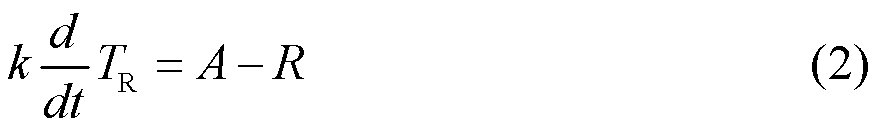where k is a constant that converts between temperature and energy per unit area. Substituting for TR using Eq. (5) of post 8, which introduces λSB, *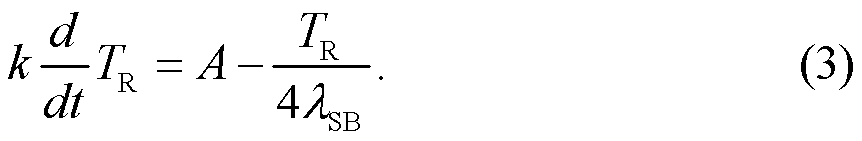Rearranging the constants,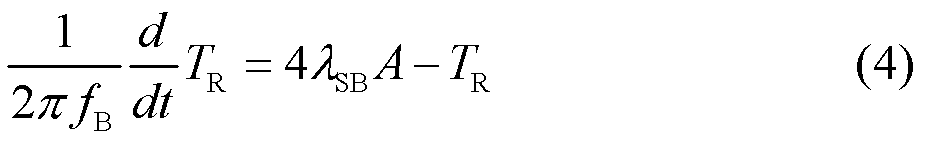where the “break frequency” isEq. (4) is the differential equation of a low pass filter of first order (like a simple RC filter in electronics). Its effect is simply to smooth out TR, as it mimics A. Because the rate of change of the radiating temperature TR is proportional to how different TR is from λSBA, it mimics the ASR but with a distorted lag — because the closer TR is to λSBA the less TR changes, and TR changes in the direction that minimizes its difference with λSBA. Thus Eq. (4) describes the thermal inertia of the Earth, or how the radiating temperature tracks the ASR. (Note that the amount of lag depends on frequency, but is not proportional to frequency (see Eq. (10) below), so in general the signal in the ASR is distorted and smoothed out by the time it gets to the OLR. It is only a simple lag if the ASR is a pure sinusoid.)

Consider a system whose input is the ASR, whose output is the radiating temperature, and where the relationship between them is as per Eq. (4). (The Earth from space would appear as such a system.) That equation is a linear differential equation, so the system is linear and invariant. Hence if the input to the system is a sinusoid then the output is a sinusoid at the same frequency. Letting the ASR input A be the sinusoidthe output  is therefore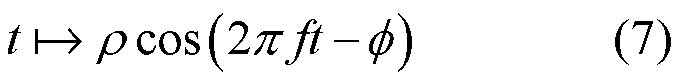for some amplitude change ρ and phase change φ. So, by Eq. (4),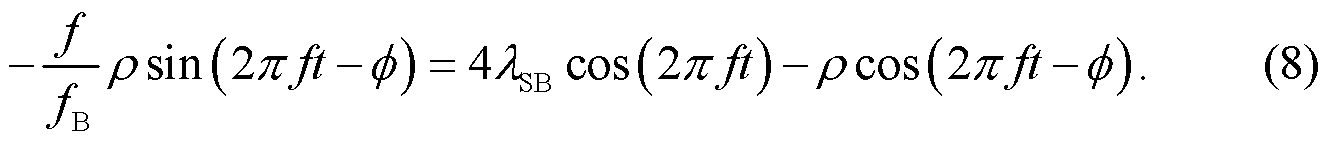Expanding the trigonometric functions then equating the coefficients of cos(2πft) and sin(2πft) gives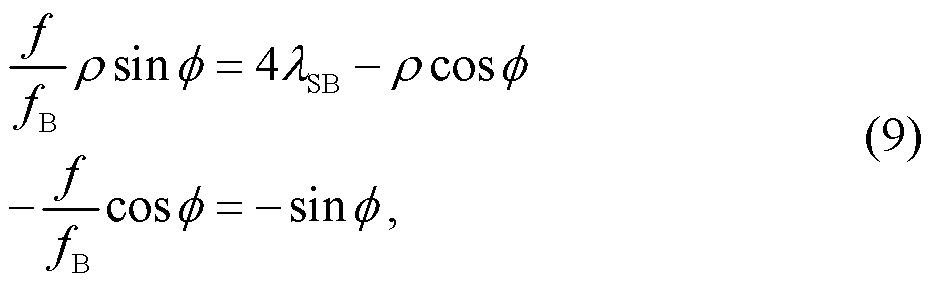to which the solution is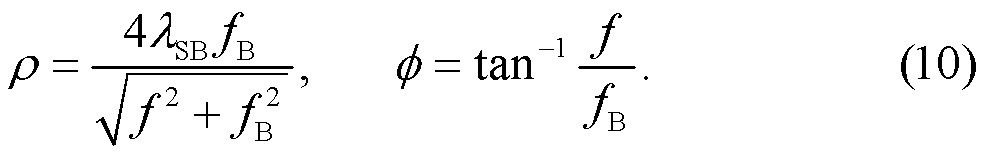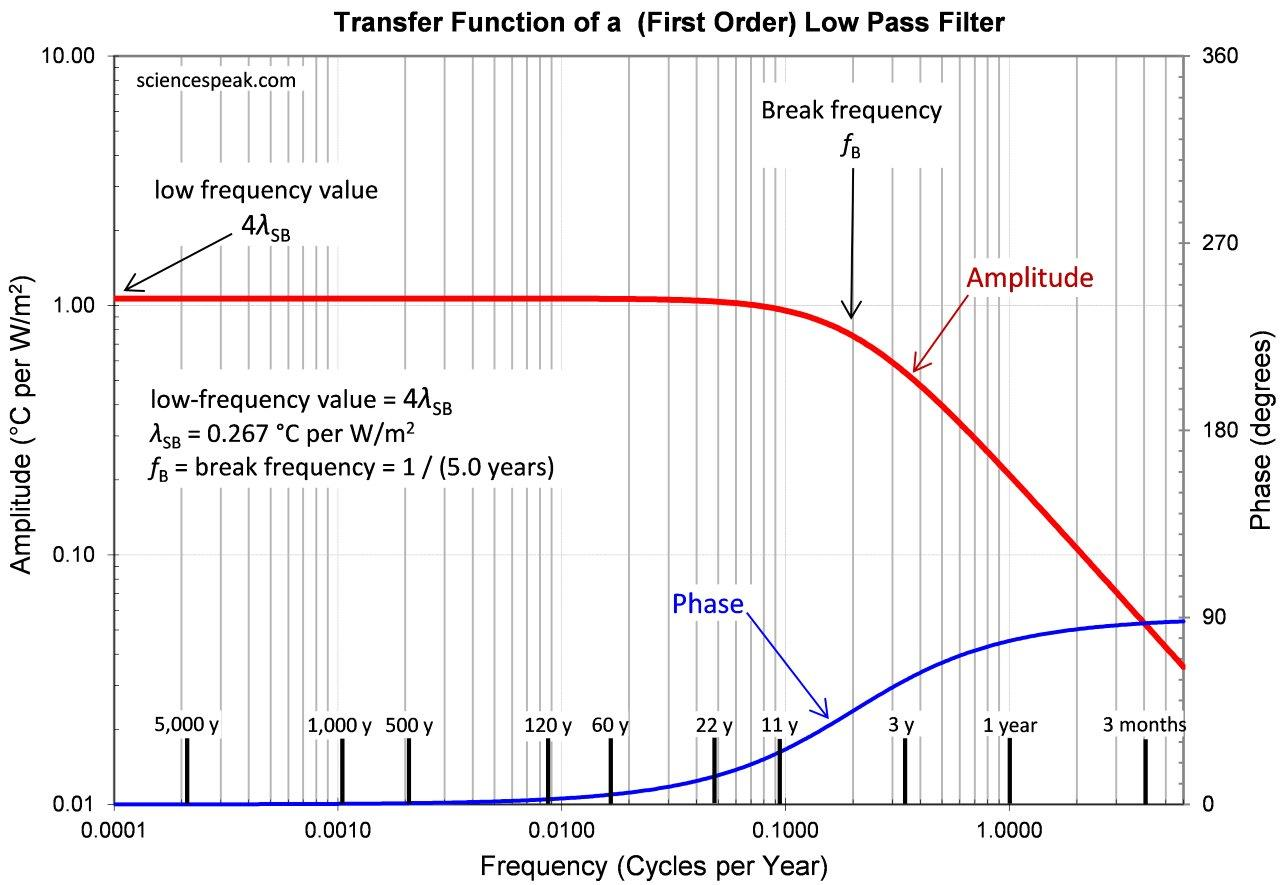Figure 1: The low pass filter of Eq. (10), in the frequency domain. The red and blue lines are from the two parts of Eq. (10).

Eq. (10) shows that slow changes in the ASR are faithfully echoed by the radiating temperature, because for frequencies well below fB the ratio of the output amplitude to the input amplitude is a constant λSB. But quick changes in the ASR are weakly echoed in the radiating temperature, because for a frequency f well above fB the ratio of the output amplitude to the input sinusoid is a tiny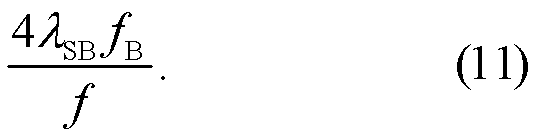The switchover between copying the input sinusoid (low frequencies) and increasingly attenuating it (high frequencies) occurs around the break frequency fB. This is the behavior one would expect due to the thermal inertia of the Earth — the temperature of the Earth tracks slow changes in the ASR but increasingly isn’t quick enough to track faster changes.

Later in this series, when we observe the empirical transfer function from total solar irradiation (TSI) to surface temperature, we find that 1/fB is either about five years or about 20 years, depending on whether it is on the high-frequency or low-frequency side of the notch (the data generally fits slightly better on the high-frequency side). This corresponds to a step response whose time constant  is about 1/(2πfB), or about 0.8 years or 3.2 years. That is, after a step change in the ASR level it takes the surface temperature about 0.8 years or about 3.2 years to reach 63% (or 1- e-1) of its new corresponding level, and about  1.8 years or 7.4 years to reach 90% of its new corresponding level. Clearly this time lag reflects the thermal inertia of the atmosphere and land, and maybe some upper layer of the ocean (i.e. the outer boundary of the climate system), but not the remainder of the ocean.

For short excursions around the Earth’s current position on the Stefan-Boltzmann curve, by Eq. (5) of post 8For transitions between steady states ΔR is equal to ΔA. Hence for small transitions between steady states,Obviously enough, the increase in radiating temperature is the product of the increase in ASR and the Stefan-Boltzmann sensitivity (SBS).

* 17 Oct 2015: Correction to post. Originally Eq. (3) omitted the factor of 4 (in the denominator of the last term). Correcting this error changed some scaling factors in equations, but not the character of the post or anything of consequence. The correction caused λSB to be replaced by 4λSB in Eq.s (4) through (10), and changed fB from (2πSB)-1 to (8πSB)-1. Because it comes from comparing  fB to the empirical transfer function, the estimated value of fB and the time constants estimated from fB were not affected by the correction. The logic of the “steady state” section, leading to Eq. (13), as initially posted was incorrect, and happened to give the correct result only because of the error in Eq. (3). Fixing Eq. (3) exposed this faulty logic. The “steady state” section has been rewritten using different reasoning, but the conclusion, namely Eq. (13), is the same as before the correction.

VN:F [1.9.22_1171]